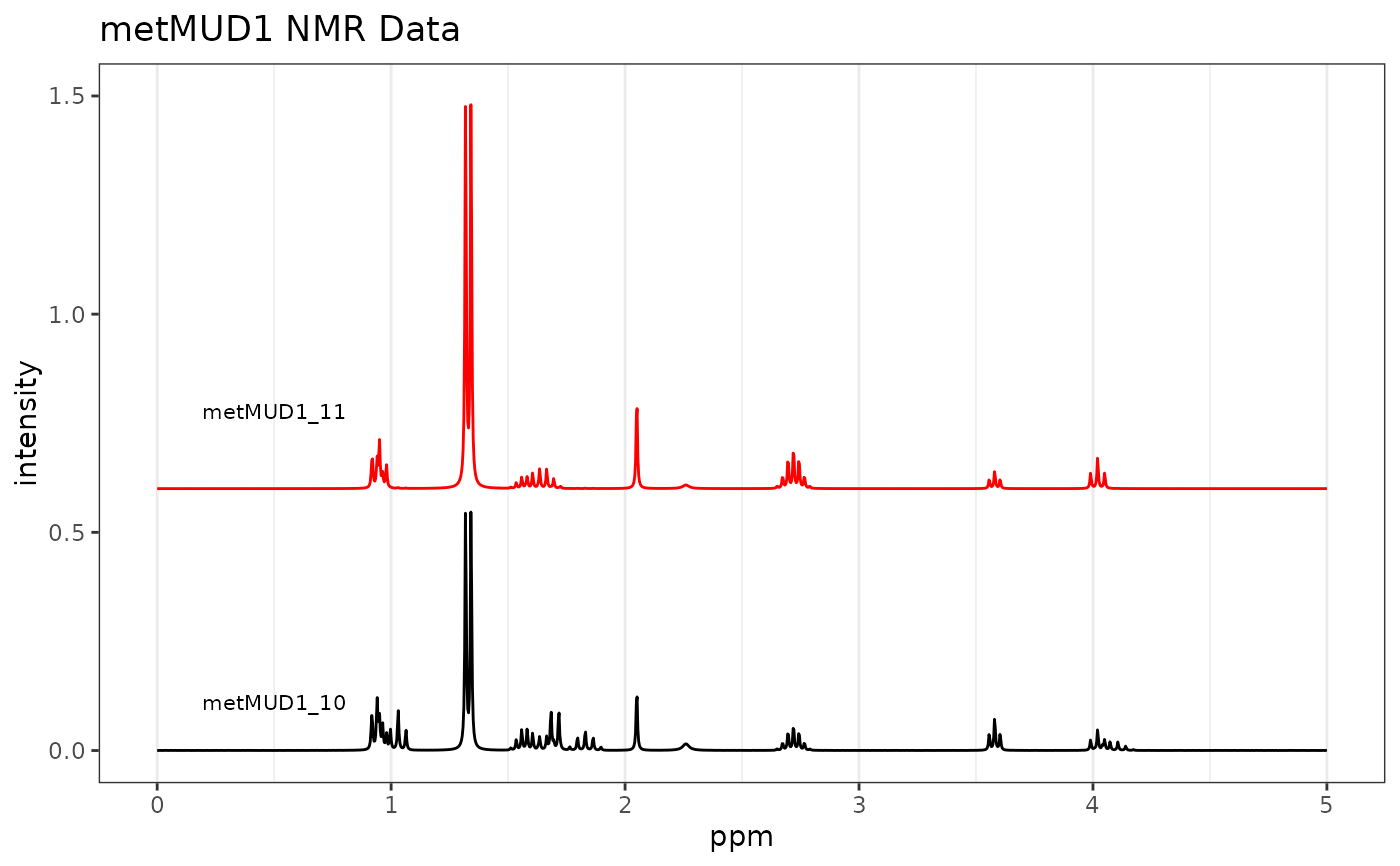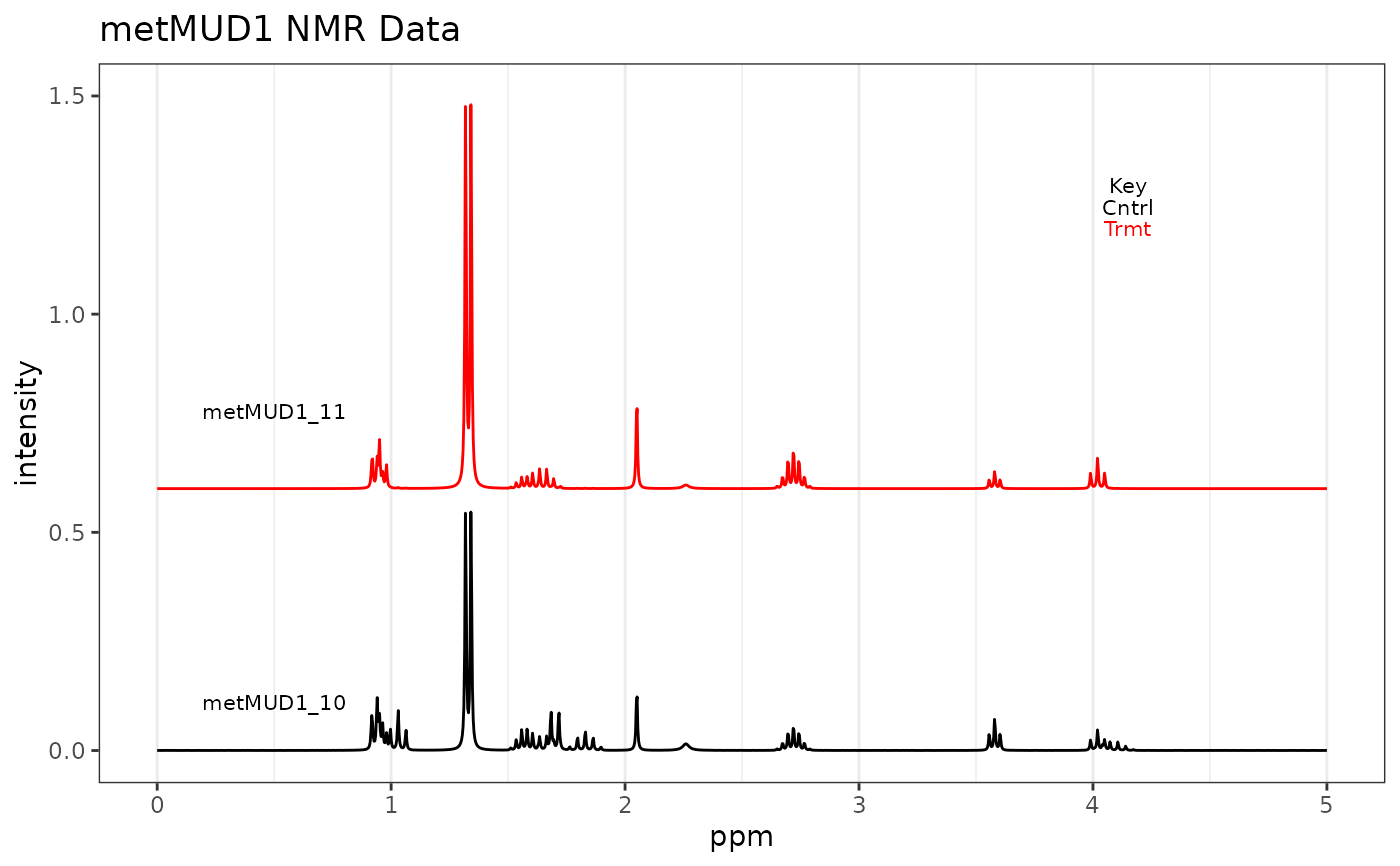Plots the spectra stored in a Spectra object. Spectra may be plotted offset or stacked. The vertical scale is controlled by a combination of several parameters.

plotSpectra(
spectra,
which = c(1),
yrange = range(spectra$data), offset = 0, amplify = 1, lab.pos = mean(spectra$freq),
showGrid = TRUE,
leg.loc = "none",
...
)

## Arguments

spectra

An object of S3 class Spectra().

which

An integer vector specifying which spectra to plot, and the order.

yrange

A vector giving the limits of the y axis desired, for instance c(0, 15). This parameter depends upon the range of values in the stored spectra and defaults to the height of the largest peak in the data set. Interacts with the next two arguments, as well as the number of spectra to be plotted as given in which. Trial and error is used to adjust all these arguments to produce the desired plot.

offset

A number specifying the vertical offset between spectra if more than one is plotted. Set to 0.0 to overlay the spectra.

amplify

A number specifying an amplification factor to be applied to all spectra. Useful for magnifying spectra so small features show up (though large peaks will then be clipped, unless you zoom on the x axis).

lab.pos

A number (in frequency units) giving the location of a label for each spectrum. Generally, pick an area that is clear in all spectra plotted. If no label is desired, set lab.pos = "none".

showGrid

Logical. Places light gray vertical lines at each tick mark if TRUE.

leg.loc

Either a list with elements x and y, or a string like 'topright'. Values in a list should be on [0,1], i.e. the lower left of the plot area is 0,0 and the upper right is 1,1. Allowed string values are those described in graphics::legend() under 'Details'. A value of 'none' is acceptable as well.

...

Parameters to be passed to the plotting routines. Applies to base graphics only.

## Value

The returned value depends on the graphics option selected (see GraphicsOptions()).

• base: None. Side effect is a plot.

• ggplot2: The plot is displayed, and a ggplot2 object is returned if the value is assigned. The plot can be modified in the usual ggplot2 manner.

plotSpectraJS for the interactive version. See GraphicsOptions for more information about the graphics options. Additional documentation at https://bryanhanson.github.io/ChemoSpec/

## Author

Bryan A. Hanson (DePauw University), Tejasvi Gupta.

## Examples

# This example assumes the graphics output is set to ggplot2 (see ?GraphicsOptions).
library("ggplot2")
data(metMUD1)

p1 <- plotSpectra(metMUD1, which = c(10, 11), yrange = c(0, 1.5),
offset = 0.06, amplify = 10, lab.pos = 0.5)
p1 <- p1 + ggtitle("metMUD1 NMR Data")
p1# Add a legend at x, y coords
p2 <- plotSpectra(metMUD1, which = c(10, 11), yrange = c(0, 1.5),
offset = 0.06, amplify = 10, lab.pos = 0.5, leg.loc = list(x = 0.8, y = 0.8))
p2 <- p2 + ggtitle("metMUD1 NMR Data")
p2